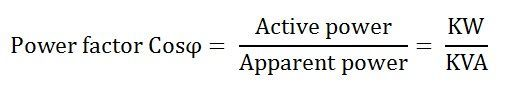# Power Triangle

Power Triangle is the representation of a right angle triangle showing the relation between active power, reactive power and apparent power.

When each component of the current that is the active component (Icosϕ) or the reactive component (Isinϕ) is multiplied by the voltage V, a power triangle is obtained shown in the figure below:The power which is actually consumed or utilized in an AC Circuit is called True power or Active Power or real power. It is measured in kilowatt (kW) or MW.

The power which flows back and forth that means it moves in both the direction in the circuit or reacts upon it, is called Reactive Power. The reactive power is measured in kilovolt-ampere reactive (kVAR) or MVAR.

The product of root mean square (RMS) value of voltage and current is known as Apparent Power. This power is measured in KVA or MVA.

The following point shows the relationship between the following quantities and is explained by graphical representation called Power Triangle shown above.

• When an active component of current is multiplied by the circuit voltage V, it results in active power.it is this power which produces torque in the motor, heat in the heater, etc. This power is measured by the wattmeter.
• When the reactive component of the current is multiplied by the circuit voltage, it gives reactive power. This power determines the power factor, and it flows back and forth in the circuit.
• When the circuit current is multiplied by the circuit voltage, it results in apparent power.
• From the power triangle shown above the power, the factor may be determined by taking the ratio of true power to the apparent power.As we know simply power means the product of voltage and current but in AC circuit except for pure resistive circuit there is usually a phase difference between voltage and current and thus VI does not give real or true power in the circuit.

### 6 thoughts on “Power Triangle”

1. Ryakela Mallesh

How you know the reactive power in a power traingle is lagging or leading ?

1. Since every circuit/load has got some kind of resistance due to the resistivity of the wires, in AC the resistance is called impedence that is inclusive of resistance and reactance. If the load is predominantly RL (an inductive reactance) then the current will lag the voltage. If the load is predominantly RC the current will lead the voltage.

2. Your explanations are most precise and comprehensive I’ve ever seen. Some times They are better than Wikipedia. But if you add some high quality ANIMATIONS alongside the diagrams like some Wikipedia articles to your explanations it would be much more BEAUTIFUL.

3. Dear sir, I heard that installing a single phase AC capacitor in 3 pin socket can save cost of electricity bill payment… Is that true? Thanks